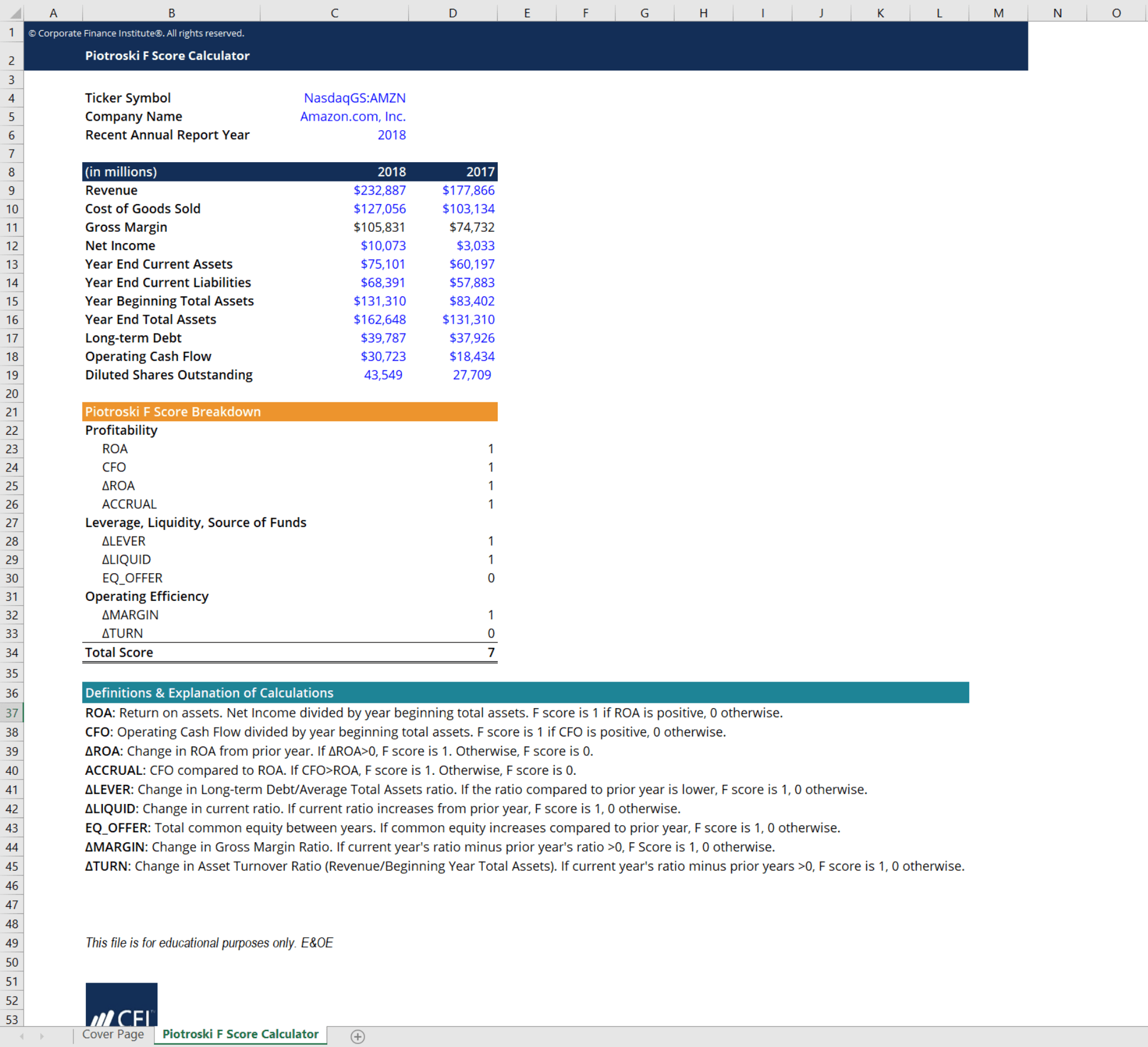# Piotroski F Score Calculator

## Piotroski F Score Calculator

This Piotroski F score calculator will help you assess the strength of a company’s financial state. The Piotroski F score uses nine factors taken from a firm’s financial statements. Using these factors, it calculates a measure of the strength of a firm’s financial position.

Here is a quick preview of CFI’s Piotroski F score calculator:### Piotroski F Score Factors

CFI’s Piotroski F score calculator can be used to assess a company’s financial strength by looking at nine factors. A score of either 0 or 1 is rewarded for each of these factors, depending on whether it has been fulfilled or not. The higher the score, the more reliable a stock is to invest in. These factors are categorized into three different sources of financial strength that analysts like to look at.

#### Profitability

ROA: Return on assets. Net Income divided by year beginning total assets. F score is 1 if ROA is positive, 0 otherwise.

CFO: Operating cash flow divided by year beginning total assets. F score is 1 if CFO is positive, 0 otherwise.

∆ROA: Change in ROA from the prior year. If ∆ROA > 0, F score is 1. Otherwise, F score is 0.

ACCRUAL: CFO compared to ROA. If CFO > ROA, F score is 1. Otherwise, F score is 0.

#### Leverage, Liquidity, and Source of Funds

∆LEVER: Change in long-term debt/average total assets ratio. If the ratio compared to the prior year is lower, F score is 1, 0 otherwise.

∆LIQUID: Change in current ratio. If the current ratio increases from the prior year, F score is 1, 0 otherwise.

EQ_OFFER: Total common equity between years. If common equity increases compared to prior year, F score is 1, 0 otherwise.

#### Operating Efficiency

∆MARGIN: Change in gross margin ratio. If the current year’s ratio minus prior year’s ratio > 0, F Score is 1, 0 otherwise.

∆TURN: Change in asset turnover ratio (revenue/beginning year total assets). If current year’s ratio minus prior years > 0, F score is 1, 0 otherwise.

CFI’s Piotroski F score calculator uses all of these factors to evaluate a company. Check out our template to conduct your own analysis on a company’s financial status!

### More Free Templates

For more resources, check out our business templates library to download numerous free Excel modeling, PowerPoint presentation, and Word document templates.

• Excel Modeling Templates
• PowerPoint Presentation Templates
• Transaction Document Templates
• CFI Template Marketplace

### Financial Analyst Certification

Become a certified Financial Modeling and Valuation Analyst (FMVA)® by completing CFI’s online financial modeling classes and training program!